# Python入门(二)——IDE选择PyCharm，输入和输出，基础规范，数据类型和变量,常量，字符串和编码，格式化Python入门(二)——IDE选择PyCharm，输入和输出，基础规范，数据类型和变量,常量，字符串和编码，格式化http://www.bieryun.com/2609.html## 一.IDE选择PyCharm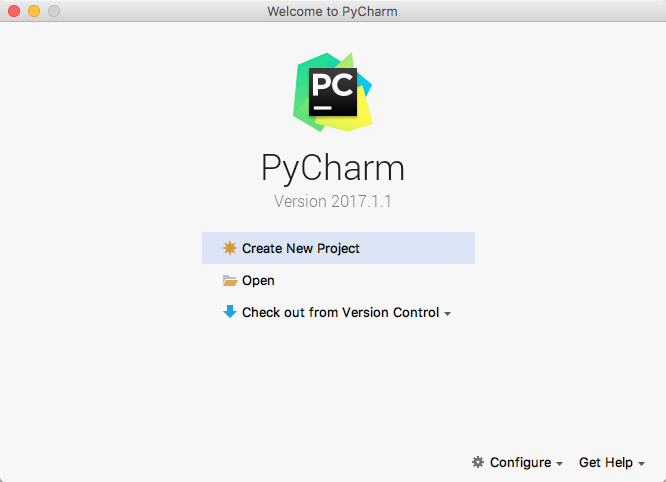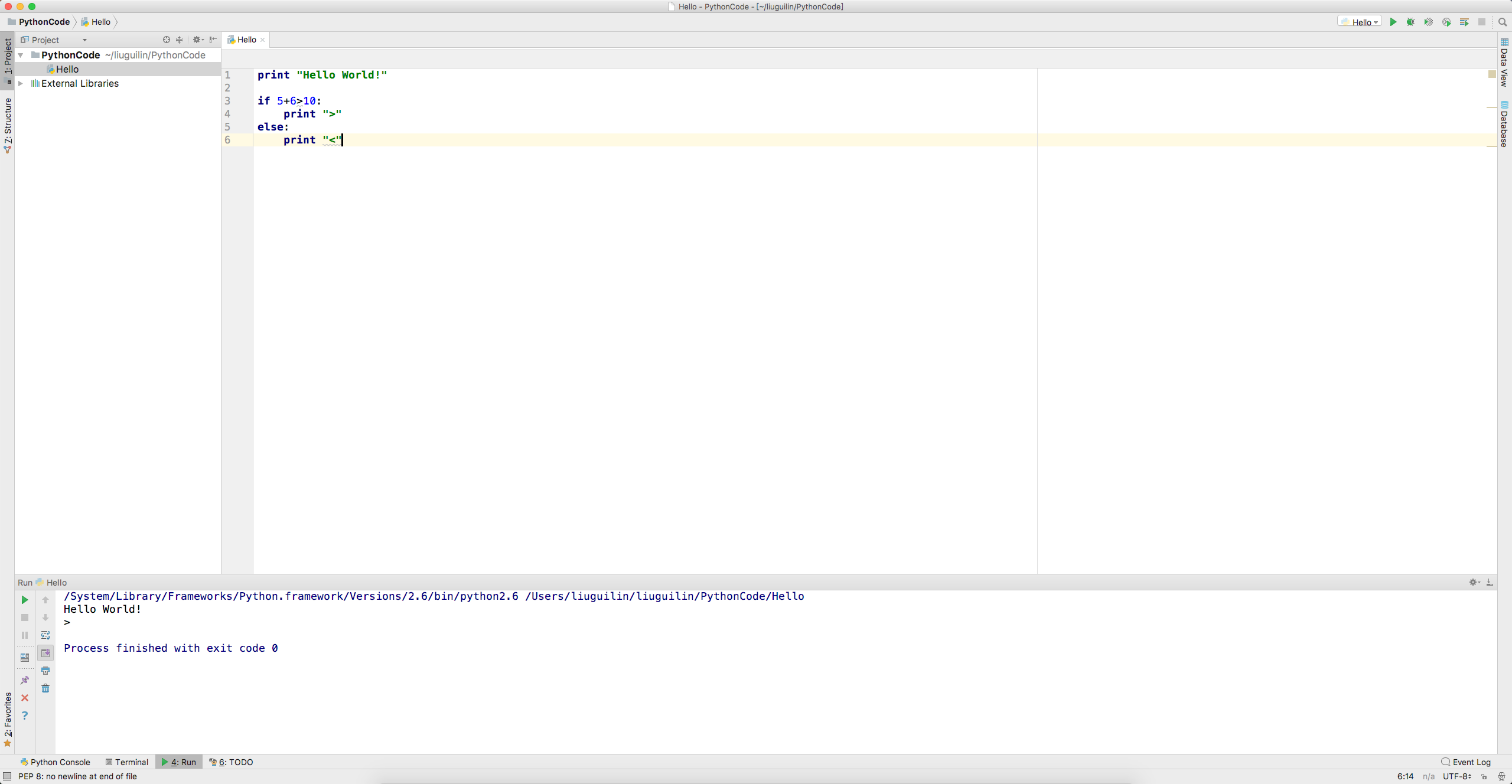## 二.输入和输出

``````print "Hello World!"

print "Hello", "World!"

print 100 + 200

print 100 > 200

print 100 < 200``````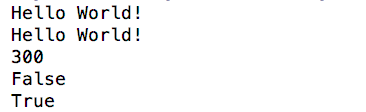ok,输出没啥说的，我们来看下输入，输入的话其实py有一个特定的函数，那就是raw_input(),我们来看下实际的案例

``````name = raw_input("Please enter your name \n")raw_input和print是在命令行下面最基本的输入和输出，但是，用户也可以通过其他更高级的图形界面完成输入和输出，比如，在网页上的一个文本框输入自己的名字，点击“确定”后在网页上看到输出信息。这些都是后话了。

## 三.基础规范

``````# print absolute value of an integer:
a = 100
if a >= 0:
print a
else:
print -a``````

## 四.数据类型和变量,常量

• 整数

python可以处理任意的整数，也包括附属，1，100 ，-20等，计算机由于是二进制有时候用十六进制表示整数更加的方便，十六进制使用0x前缀和0-9，a-f表示，比如0xff00,0xdf522等

• 浮点数

• 字符串

``print "I\'m OK!"``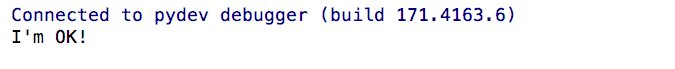``````print "Im\OK"

print "Im\\OK"

print "Im\nOK"

print "Im\tOK"``````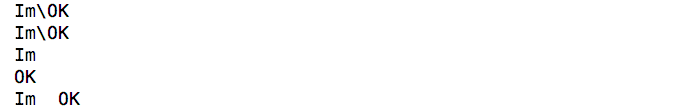``````print '\\\t\\'

print r'\\\t\\'``````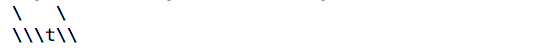``````print '''line1
line2
line3'''``````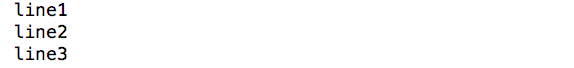• 布尔值

``````print 100 > 200

print 100 < 200``````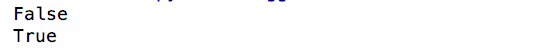and运算是与运算，只有所有都为True，and运算结果才是True：

``````print True and True

print True and False

print False and False``````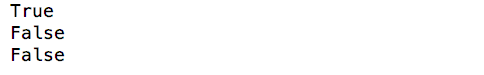or运算是或运算，只要其中有一个为True，or运算结果就是True：

``````print True or True

print True or False

print False or False``````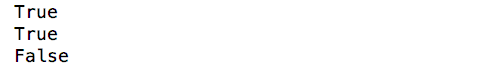not运算是非运算，它是一个单目运算符，把True变成False，False变成True：

``````print not True

print not False``````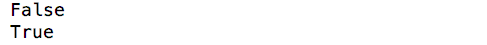• 空值

• 变量

``a * 4 = 12``

``a = 1``

``````# a是整数
a = 123
print a

# a变为字符串
a = 'ABC'
print a``````

``a = 'ABC'``

• 1.在内存中创建’ABC’的字符串
• 2.在创建一个名为a的变量，并把他指向’ABC’

``````a = "ABC"

b = a;

print b``````

• 常量

``````PI = 5555

print PI``````

## 五.字符串和编码

``````print ord('A')

print chr(65)``````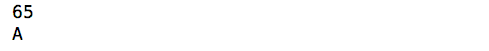``print u'刘桂林'``

``````print u'ABC'.encode('utf-8')

print u'刘桂林'.encode('utf-8')``````

``````print len("ABC")

print len(u"刘某人程序员")``````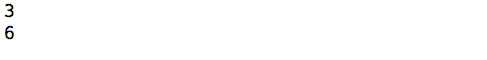``#coding:utf-8``

## 六.格式化

``亲爱的xx你好，今天是xxx,你还剩下xx天还款``

``print '亲爱的%s,你好,今天是%s,你还剩下%d天还款' % ('刘桂林', '2017年4月27日', 3)``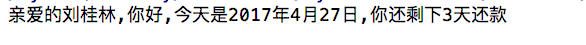• %d 整数
• %f 浮点数
• %s 字符串
• %x 十六进制整数• 回顶